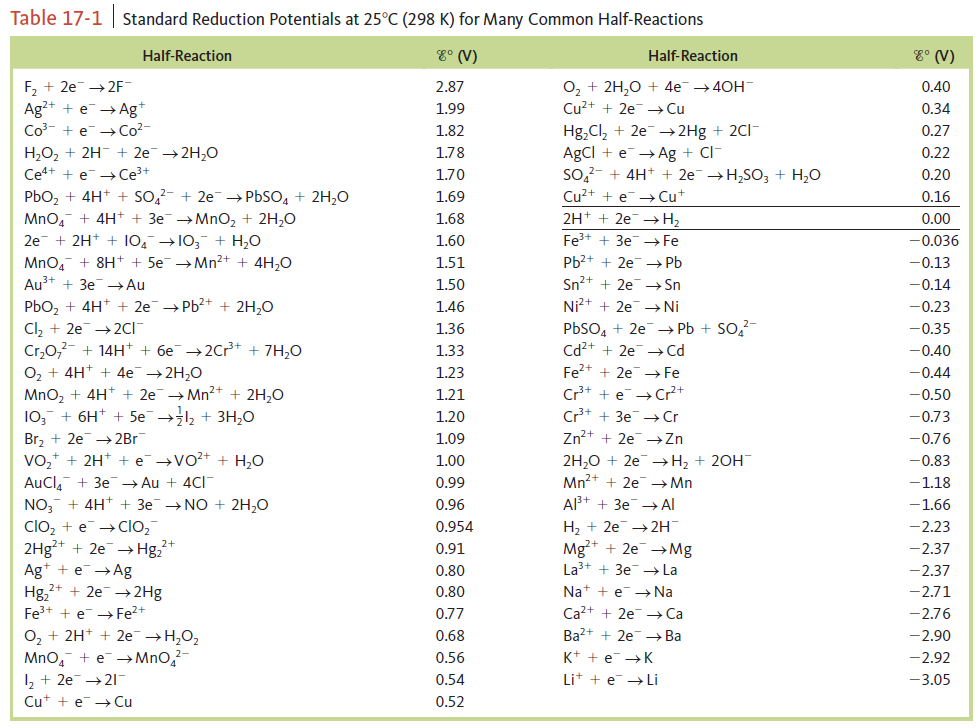# Problem: Calculate E° values for the following cells. Which reactions are spontaneous as written (under standard conditions)? Balance the equations. Standard reduction potentials are found in Table 17‑1.b. MnO4- (aq) + F - (aq) → F2 (aq) + Mn2+ (aq)

###### FREE Expert Solution

When a balanced reaction is given, identify the anode and the cathode half-reactions by determining the changes in oxidation states of each species based on the given reaction:

Recall:

Lose               Gain
Electron         Electrons
Oxidation       Reduction

cathode → reduction → oxidation number decreases

anode → oxidation → oxidation number increases

For ions: charge of the ion = oxidation number
For neutral atoms/compound: oxidation number = 0

Determine the half-cell potentials (refer to the Standard Reduction Potential Table)

F2 + 2 e- → 2 F-                                                      E°cell = 2.87 V

MnO4- + 8H+ + 5 e- → Mn2+ + 4 H2O                     E°cell = 1.51 V

92% (34 ratings)###### Problem Details

Calculate E° values for the following cells. Which reactions are spontaneous as written (under standard conditions)? Balance the equations. Standard reduction potentials are found in Table 17‑1.

b. MnO4- (aq) + F (aq) → F2 (aq) + Mn2+ (aq)Frequently Asked Questions

What scientific concept do you need to know in order to solve this problem?

Our tutors have indicated that to solve this problem you will need to apply the Cell Potential concept. If you need more Cell Potential practice, you can also practice Cell Potential practice problems.

What professor is this problem relevant for?

Based on our data, we think this problem is relevant for Professor Randles' class at UCF.

What textbook is this problem found in?

Our data indicates that this problem or a close variation was asked in Chemistry: An Atoms First Approach - Zumdahl Atoms 1st 2nd Edition. You can also practice Chemistry: An Atoms First Approach - Zumdahl Atoms 1st 2nd Edition practice problems.